Courses

# RD Sharma Solutions -Ex-8.3, Introduction To Algebra, Class 6, Maths Class 6 Notes | EduRev

## RD Sharma Solutions for Class 6 Mathematics

Created by: Abhishek Kapoor

## Class 6 : RD Sharma Solutions -Ex-8.3, Introduction To Algebra, Class 6, Maths Class 6 Notes | EduRev

The document RD Sharma Solutions -Ex-8.3, Introduction To Algebra, Class 6, Maths Class 6 Notes | EduRev is a part of the Class 6 Course RD Sharma Solutions for Class 6 Mathematics.
All you need of Class 6 at this link: Class 6

Mark the correct alternatice in each of the following:

1. 5 more than twice a number x is written as

(a) 5 + x + 2

(b) 2x + 5

(c) 2x - 5

(d) 5x + 2

2. The quotient of x by 2 is written as

(a)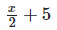(b)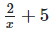(c)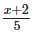(d)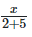Answer: (a)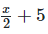3. The quotient of x by 3 is multiplied by y is written as

(a)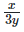(b)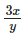(c)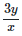(d)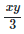Answer: (d)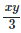4. 9 taken away from the sum of x and y is

(a) x + y - 9

(b) 9 - (x + y)

(c)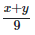(d)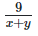Answer: (a) x + y - 9

5. The quotient of x by y added to the product of x and y is written as

(a)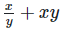(b)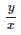+xy

(c)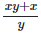(d)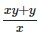Answer: (a)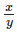+xy

6. a2b3×2ab2 is equal to

(a) 2a3b4

(b) 2a3b5

(c) 2ab

(d) a3b5

7. 4a2b3×3ab2×5a3b is equal to

(a) 60a3b5

(b) 60a6b5

(c) 60a6b6

(d) a6b6

8. if 2x2y and 3xy2 denote the length and breadth of a rectangle, then its area is

(a) 6xy

(b) 6x2y2

(c) 6x3y3

(d) x3y3

9. In a room there are x2 rows of chairs and each rows contains 2xchairs. The total number of chairs in the room is

(a) 2x2

(b) 2x4

(c) x4

(d) x44

10. a3×2a2b×3ab5 is equal to

(a) a6b6

(b) 23a6b6

(c) 6a6b6

(d) None of these

103 docs

,

,

,

,

,

,

,

,

,

,

,

,

,

,

,

,

,

,

,

,

,

,

,

,

,

,

,

,

,

,

;